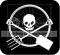## Monday, 5 March 2012

### calculus in the gardenThe other day I got a chance to use some calculus for real-world stuff.

So our yard is one of those that floods when it rains. It's clay and it has trough shapes. There is one main trough shape and I thought that I would like to get it filled up with soil. So how much soil would I need?

Well I knew I could solve this with an integral. So I went ahead and assumed the curve of the trough was parabolic. Who knows what it was but this was a fair guess.

I went out and measured the depth of the trough and gauged its length. That gave me my parabolic function. It was something like y=-(13/(36^2))x^2+13. The integral of this multiplied by the trough's length gave me the volume of the trough. So it turns out I need around 122 cubic feet of soil.

Turns out that costs somewhere around \$200-\$500. So looks like I'm keeping the trough. :D